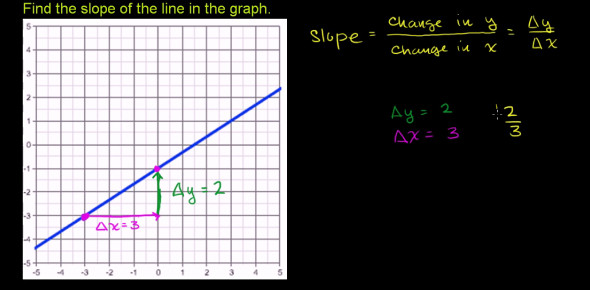# Slope Of A Line Quiz! Trivia Test

9 Questions | Total Attempts: 122Settings• 1.
The _____________ is the horizontal distance between two points.
• 2.
The _______________ is the vertical distance between two points.
• 3.
Find the slope of the line.
• 4.
Find the slope of the line.
• 5.
Find the slope of the line.
• 6.
Find the slope of the line.
• 7.
Find the slope of the line.
• 8.
Find the slope of the line.
• 9.
Find the slope of the line.
Related TopicsBack to top